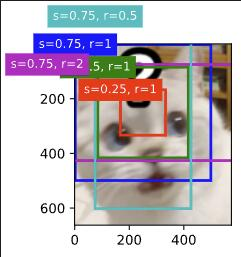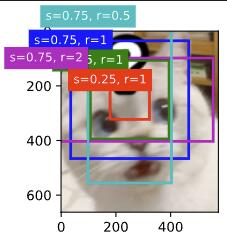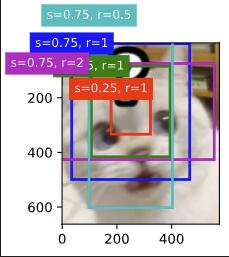# 锚框

（20个字符）

s指缩放比 r指宽高比 w,h原图像宽和高

h’ = h * s / sqrt(r)

w’ / w = s * sqrt(r)
h’ / h = s / sqrt(r)

（代码太难懂，评论区各抒己见，公式都不太一样，官方能否给出锚框宽度和高度公式的由来，不然不清不楚的，大家都在猜不同的r和s可能是什么意思。）

``````w = torch.cat((size_tensor * torch.sqrt(ratio_tensor),
sizes * torch.sqrt(ratio_tensor[1:])))\
* in_height / in_width  # 处理矩形输入
``````

``````w = torch.cat((size_tensor * torch.sqrt(ratio_tensor),
sizes * torch.sqrt(ratio_tensor[1:])))\
* in_height / in_width
h = torch.cat((size_tensor / torch.sqrt(ratio_tensor),
sizes / torch.sqrt(ratio_tensor[1:])))
``````

1、原图坐标进行了归一化，描框坐标显然同样需要归一化，归一化后显然没有 * in_height / in_width这个操作；
2、我们认为size_tensor=1且ratio_tensor=1时生成的描框为基准描框，当w中没有* in_height / in_width这一项时，基准描框的实际尺寸为原图像的尺寸，此时就不能保证我们的基准描框实际尺寸一定为正方形。当原图为正方形时，基准描框为正方形；当原图不为正方形时，我们仍然想要基准描框为正方形(单纯的人为设置)，因此需要在w上* in_height / in_width，这时当我们看上述公式，s=1，r=1，对w和h同时乘in_width后，基准描框的长和宽就均为in_height了，满足正方形

``````    w =t.cat((size_tensor*t.sqrt(ratio_tensor),sizes*t.sqrt(ratio_tensor[1:])))
h = t.cat((size_tensor/t.sqrt(ratio_tensor),sizes/t.sqrt(ratio_tensor[1:])))/in_height*in_width
``````

1. 对w进行归一：2.对h进行归一：3.什么都不干：1 Like

Anchor 的两种编程实现 - xinet - 博客园 (cnblogs.com)